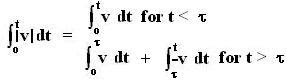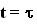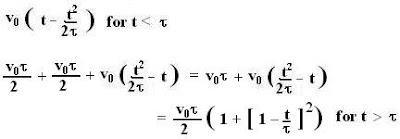## Tuesday, July 17, 2007

### Irodov Problem 1.21

The position of the particle as a function of time is given byThe exact values of the for a and b parts can be solved by using the above equation.
c) the distance covered is actuallysince the velocity changes its sign at.

Thus, the distance is given by,The exact numerical values can be obtained by substituting in the above equation.